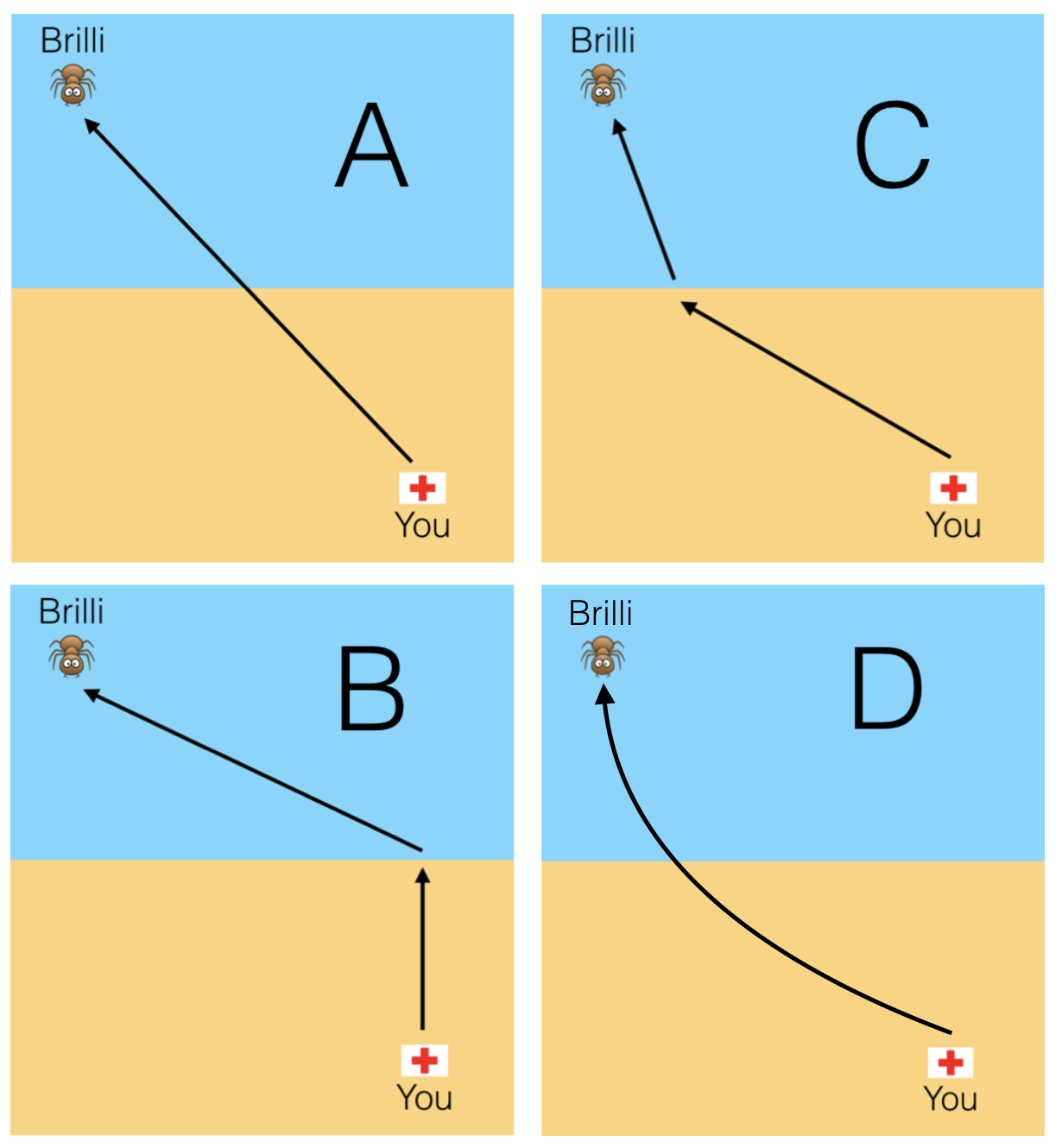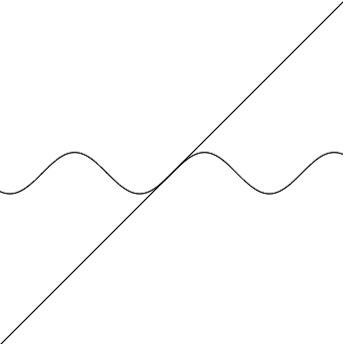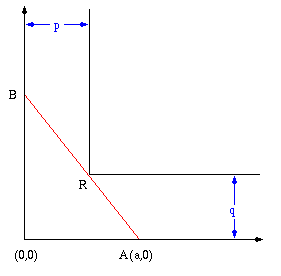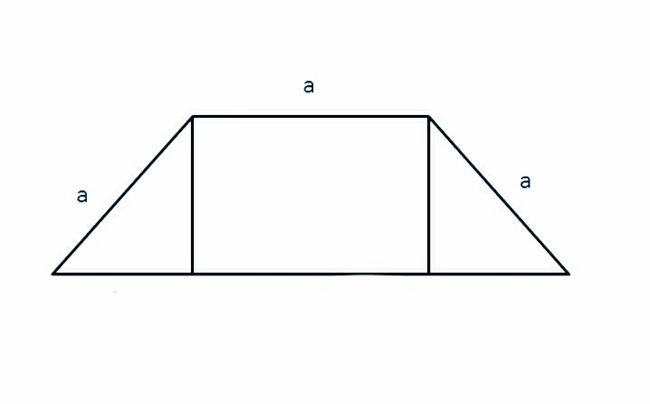Calculus

Extrema: Level 2 Challenges

You have just started your summer job as a lifeguard at the beach, which has oversized ants leisurely swimming. You are on top of your lifeguard stand and notice that Brilli the ant is making distress signals some distance offshore, and you want to travel to Brilli in the quickest way possible.Knowing that you travel faster running over sand than you do swimming through water, which of the above best illustrates the quickest path to take?

Assume that the water is still, with no currents.Is $f(x)= x + \sin x$ an injective function?What is the approximate length of the longest ladder (in feet) you can carry horizontally around the corner of the corridor shown here?

$AB$ is the ladder and the coordinate of $R$ is $(8,6)$.A right triangle $ABC$ with side $AB = x$ and $BC = 12$ intersects a square with side $AB$ at the point $D$. Assuming $x$ is variable, find the value of $x\le 12$ that maximizes the shaded portion of the triangle.What is the maximum​ area of a trapezium which has 3 sides of length $\color{#3D99F6} a$?

×